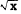Custom SearchTRANSLATING FORMULAS Thus far, we have been concerned primarily with reducing verbal rules or statements to formula form. It is also necessary to be able to do the reverse, and translate a formula into words. Technical publications frequently take advantage of the fact that it is more convenient to write formulas than longhand rules. Understanding is hampered if we are not able to translate these formulas into words. As an example of translation, we may translate the formula V = lwh into words, with the literal factors representing words as follows:             V = volume of a rectangular solid             l = length             w = width             h = height This produces the following translation: The volume of a rectangular solid equals the length times the width times the height. As a second example, we translate the algebraic expression 2- 4 into words as follows: Twice the square root of a certain number, minus 4. Practice problems. Translate each of the following expressions into words. 1. PV = k, where P represents pressure of a gas and V represents volume. (Assume constant temperature .) 2. x = y + 4, where x and y are numbers. 3. A = LW, where A is the area of a rectangle, L is its length, and W is its width. 4. d = rt, where d is distance, r is rate, and t is time. Answers: 1. The pressure of a gas multiplied by its volume is constant, if the temperature is constant. 2. A certain number, x, is equal to the sum of another number, y, and 4. 3. The area of a rectangle is equal to the product of its length times its width. 4. Distance is equal to rate multiplied by time. GRAPHING FORMULAS We have seen that the formula is an equation. Since all formulas are equations they may be graphed. Graphs of formulas have wide use in the Navy in such fields as electronics and engineering. In practical applications it is often convenient to derive information from graphs of formulas rather than from formulas directly. As an example, suppose that a fuel costs 30 cents per gallon. The formula for the cost in dollars of n gallons is C = 0.30n We see that this is a linear equation, the resulting curve of which passes through the origin (no constant term). Since we are interested only in positive values, we can eliminate three quadrants of the graph and use only the first quadrant. We already know one point on the graph is (0,0). We need plot only one other point to graph the formula. The result is shown In figure 14-4.Figure 14-4.-Graph for the formula C = 0.30n. We may read the cost directly from the graph when the number of gallons is known, or the number of gallons when the cost is known. For instance, if 5-1/2 gal are sold, find 5-1/2 on the gallons scale and follow the vertical line from that point to the point where it intersects the graph of the formula. From this point, follow the horizontal line to the cost scale. The horizontal line intersects ‘the cost scale at 1.65. Therefore the cost of 5-l/2 gal is \$1.65. Likewise, to answer the question, "How many gallons may be bought for \$1.27," we would enlarge the graph enough to estimate to the exact cent. Then we would follow a horizontal line from 1.27 on the cost scale to the formula graph and follow a vertical line from that point to the gallons scale. Thus, 4-1/4 gal may be bought for \$1.27. Plotting two formulas on the same graph may help to solve certain kinds of problems. For example, suppose that two ships leave port at the same time. One averages 10 knots and the other averages 15 knots. How far has each traveled at the end of 3 hr and at the end of 5 hr ? A graph to relate the two ships’ movements at any time can be made as follows: Let the vertical scale be in nautical miles and theFigure 14-5.-Graph of the formulas d = 10t and d = 15t. horizontal scale be in hours. The formula for the first ship’s distance related to time is         d = 10t The formula for the second ship’s distance related to time is         d = 15t We see that these formulas are linear and their curves pass through the origin. They are graphed in figure 14-5. With this graph we can now answer the questions originally posed, at a glance. Thus in 3 hr the first ship traveled 30 mi and the second traveled 45 mi. In 5 hr the first ship traveled 50 mi and the second traveled 75 mi. We could also answer such questions as: When the second ship has traveled 100 mi, how far has the other traveled? We first find the point on the graph of d = 15t where the ship has traveled 100 mi. We then follow the vertical line from that point to the point where it intersects the graph of the other formula. From the point of intersection we follow a horizontal line to the distance axis and see that the first ship has traveled about 67 mi when the second has traveled 100 mi. The foregoing examples serves to illustrate the wide variety of applications in which graphs of formulas are useful.